# 一、光晕逻辑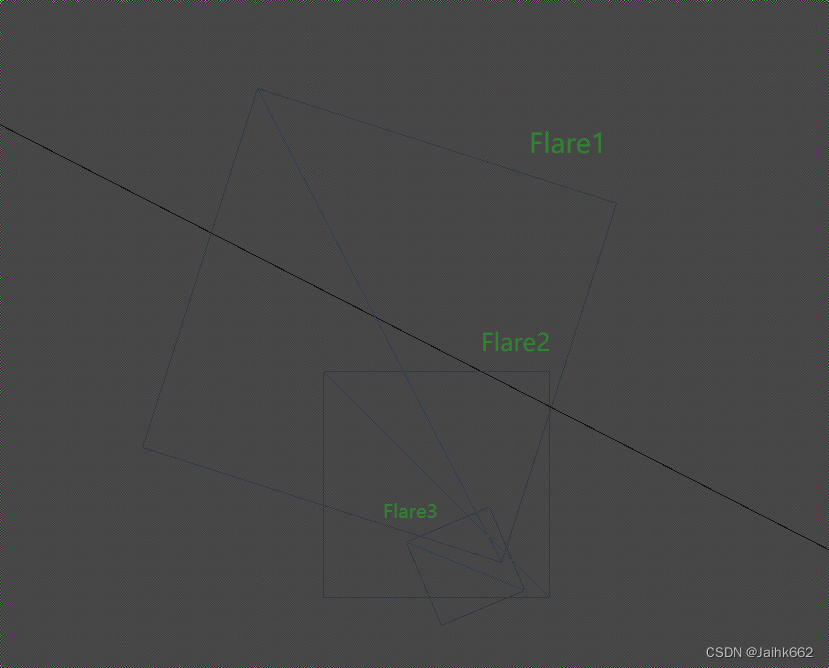## 1.1 Unity URP 光晕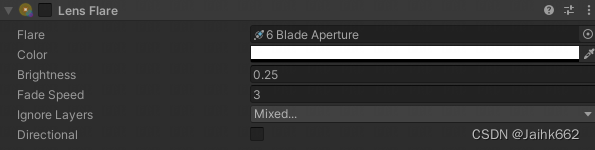## 1.2 光晕的可见性

1. 场景中的太阳可视时，会出现镜头光晕效果
2. 光晕在屏幕上的位置分布与场景中的太阳方位，和当前视角都有一定关系

``````half3 D = _ROCLightDir1;
float4 clip = TransformWorldToHClip(GetCameraRelativePositionWS(D * 10000));
float depth = LinearEyeDepth(0, _ZBufferParams);``````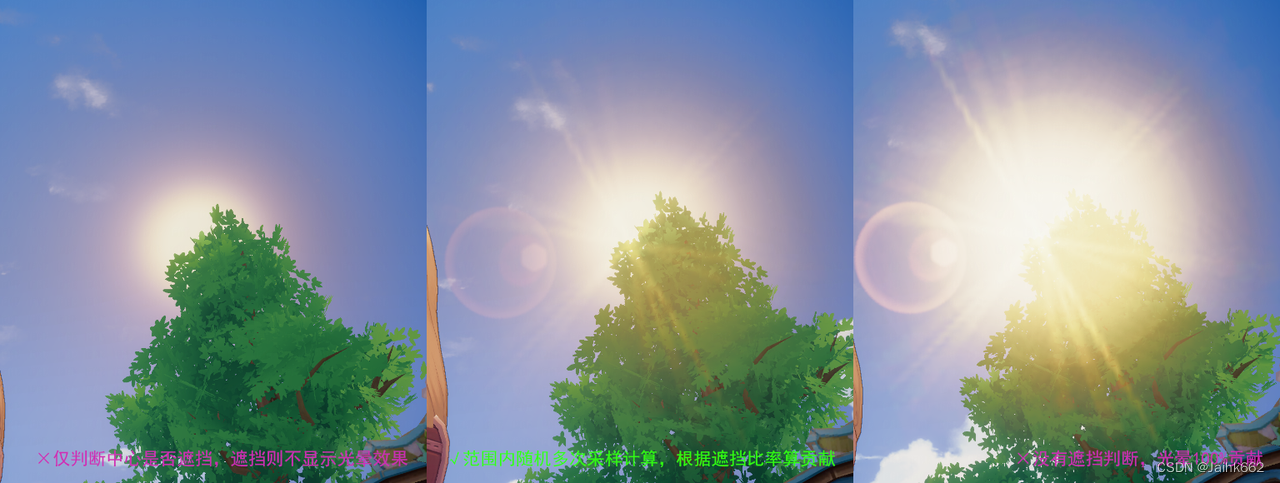``````//thanks, internets
static const uint DEPTH_SAMPLE_COUNT = 32;
static float2 samples[DEPTH_SAMPLE_COUNT] = {
float2(0.658752441406,-0.0977704077959),
float2(0.505380451679,-0.862896621227),
float2(-0.678673446178,0.120453640819),
//…… 32 组随机数，略
};

float GetOcclusion(float2 screenPos, float depth, float radius, float ratio)
{
float contrib = 0.0f;
float sample_Contrib = 1.0 / DEPTH_SAMPLE_COUNT;
float2 ratioScale = float2(1 / ratio, 1.0);
for (uint i = 0; i < DEPTH_SAMPLE_COUNT; i++)
{
float2 pos = screenPos + (samples[i] * radius * ratioScale);
pos = pos * 0.5 + 0.5;
pos.y = 1 - pos.y;
if (pos.x >= 0 && pos.x <= 1 && pos.y >= 0 && pos.y <= 1)
{
if (sampledDepth >= depth)
contrib += sample_Contrib;
}
}
return contrib;
}

vert()
{
float2 screenPos = clip.xy / clip.w;
float ratio = _ScreenParams.x / _ScreenParams.y;
float occlusion = GetOcclusion(screenPos, depth, radius, ratio);
}``````

## 1.3 Mesh 生成与着色

``````struct appdata
{
float4 vertex: POSITION;
float2 uv: TEXCOORD0;
float4 color: COLOR;

// LensFlare Data :
//      * X = RayPos
//      * Y = Rotation (< 0 = Auto)
//      * ZW = Size (Width, Height) in Screen Height Ratio
nointerpolation float4 lensflare_data: TEXCOORD1;
// World Position (XYZ) and Radius(W) :
//      * X = Near Start Distance
//      * Y = Near End Distance
//      * Z = Far Start Distance
//      * W = Far End Distance
};``````

## 1.4 后续性能优化方案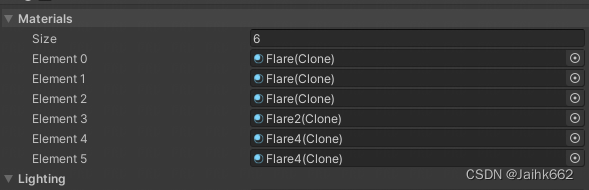# 二、手机各平台兼容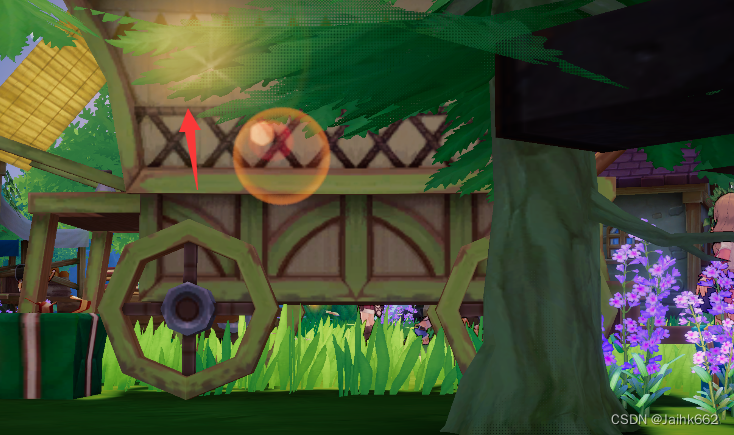## 2.1 是否当前的手机平台不支持顶点着色器采样纹理

• 但仍然需要注意的是：Unity 若要在顶点着色器中采样纹理，需要指定 mipmap 等级，这就意味着不能使用常规的 API 例如 SAMPLE_TEXTURE2D(…)，需要用 SAMPLE_TEXTURE2D_LOD(…, 0) 代替，这是因为 mipmap 的级别的选择需要在片段着色阶段才能获取

## 2.2 是否是因为指定的着色器模型，默认编译目标设置过高

``#pragma target 5.0``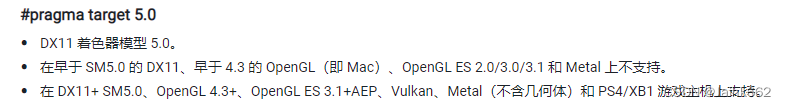## 2.3 疑似屏幕坐标计算错误

``````o.pos = TransformObjectToHClip(v.pos.xyz);
o.screenUV = ComputeScreenPos(o.pos);
half2 screenUV = i.screenUV.xy / i.screenUV.w;

### 2.3.1 ComputeScreenPos

``````float4 ComputeScreenPos(float4 positionCS)
{
float4 o = positionCS * 0.5f;
o.xy = float2(o.x, o.y * _ProjectionParams.x) + o.w;
o.zw = positionCS.zw;
return o;
}``````

``````screenUV.x = ((pos.x / w) * 0.5 + 0.5) * width;
screenUV.y = ((pos.y / w) * 0.5 + 0.5) * height;``````

Unity 为什么要这样做呢？即为什么不直接帮我们直接映射到 [0, 1]？

1. 考虑 tex2Dproj 指令，其会在对纹理采样前帮我们把参数除上一个 w 分量，这样你在 ComputeScreenPos 之后直接套用这个方法就是正确的了，只不过我们很少用这个 tex2Dproj 指令
2. 如果你要在片段着色器中采样，非常不建议在顶点着色器中就提前除以 w 分量，此会导致经过插值到片段着色器后，得到的插值结果不准确，因此最好是先插值再归一化，这是因为投影空间不是线性空间

• 如果是 Direct3D-like 平台(UNITY_UV_STARTS_AT_TOP = 1)，就需要进行翻转
• 如果是 OpenGL-like 平台(UNITY_UV_STARTS_AT_TOP = 0)，则无需翻转

## 2.4 Reversed-Z

``float depth = LinearEyeDepth(0, _ZBufferParams);``

``````#if UNITY_REVERSED_Z
float depth = LinearEyeDepth(0, _ZBufferParams);
#else
float depth = LinearEyeDepth(1, _ZBufferParams);
#endif``````